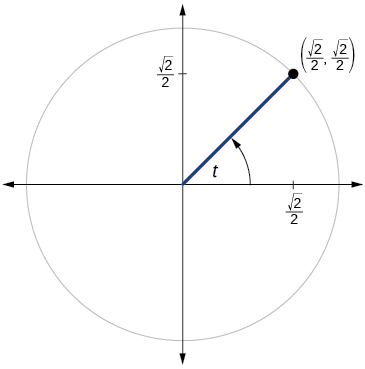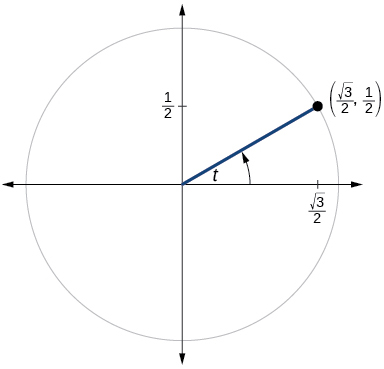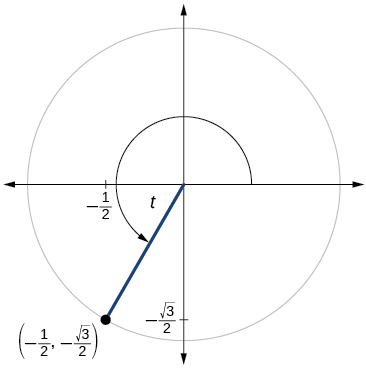# 5.3 The other trigonometric functions  (Page 7/13)

 Page 7 / 13

## Algebraic

For the following exercises, find the exact value of each expression.

$\mathrm{tan}\text{\hspace{0.17em}}\frac{\pi }{6}$

$\mathrm{sec}\text{\hspace{0.17em}}\frac{\pi }{6}$

$\frac{2\sqrt{3}}{3}$

$\mathrm{csc}\text{\hspace{0.17em}}\frac{\pi }{6}$

$\mathrm{cot}\text{\hspace{0.17em}}\frac{\pi }{6}$

$\sqrt{3}$

$\mathrm{tan}\text{\hspace{0.17em}}\frac{\pi }{4}$

$\mathrm{sec}\text{\hspace{0.17em}}\frac{\pi }{4}$

$\sqrt{2}$

$\mathrm{csc}\text{\hspace{0.17em}}\frac{\pi }{4}$

$\mathrm{cot}\text{\hspace{0.17em}}\frac{\pi }{4}$

1

$\mathrm{tan}\text{\hspace{0.17em}}\frac{\pi }{3}$

$\mathrm{sec}\text{\hspace{0.17em}}\frac{\pi }{3}$

2

$\mathrm{csc}\text{\hspace{0.17em}}\frac{\pi }{3}$

$\mathrm{cot}\text{\hspace{0.17em}}\frac{\pi }{3}$

$\frac{\sqrt{3}}{3}$

For the following exercises, use reference angles to evaluate the expression.

$\mathrm{tan}\text{\hspace{0.17em}}\frac{5\pi }{6}$

$\mathrm{sec}\text{\hspace{0.17em}}\frac{7\pi }{6}$

$-\frac{2\sqrt{3}}{3}$

$\mathrm{csc}\text{\hspace{0.17em}}\frac{11\pi }{6}$

$\mathrm{cot}\text{\hspace{0.17em}}\frac{13\pi }{6}$

$\sqrt{3}$

$\mathrm{tan}\text{\hspace{0.17em}}\frac{7\pi }{4}$

$\mathrm{sec}\text{\hspace{0.17em}}\frac{3\pi }{4}$

$-\sqrt{2}$

$\mathrm{csc}\text{\hspace{0.17em}}\frac{5\pi }{4}$

$\mathrm{cot}\text{\hspace{0.17em}}\frac{11\pi }{4}$

−1

$\mathrm{tan}\text{\hspace{0.17em}}\frac{8\pi }{3}$

$\mathrm{sec}\text{\hspace{0.17em}}\frac{4\pi }{3}$

−2

$\mathrm{csc}\text{\hspace{0.17em}}\frac{2\pi }{3}$

$\mathrm{cot}\text{\hspace{0.17em}}\frac{5\pi }{3}$

$-\frac{\sqrt{3}}{3}$

$\mathrm{tan}\text{\hspace{0.17em}}225°$

$\mathrm{sec}\text{\hspace{0.17em}}300°$

2

$\mathrm{csc}\text{\hspace{0.17em}}150°$

$\mathrm{cot}\text{\hspace{0.17em}}240°$

$\frac{\sqrt{3}}{3}$

$\mathrm{tan}\text{\hspace{0.17em}}330°$

$\mathrm{sec}\text{\hspace{0.17em}}120°$

−2

$\mathrm{csc}\text{\hspace{0.17em}}210°$

$\mathrm{cot}\text{\hspace{0.17em}}315°$

−1

If $\text{\hspace{0.17em}}\text{sin}\text{\hspace{0.17em}}t=\frac{3}{4},$ and $\text{\hspace{0.17em}}\text{\hspace{0.17em}}t\text{\hspace{0.17em}}$ is in quadrant II, find $\text{\hspace{0.17em}}\mathrm{cos}\text{\hspace{0.17em}}t,\mathrm{sec}\text{\hspace{0.17em}}t,\mathrm{csc}\text{\hspace{0.17em}}t,\mathrm{tan}\text{\hspace{0.17em}}t,\mathrm{cot}\text{\hspace{0.17em}}t.$

If $\text{\hspace{0.17em}}\text{cos}\text{\hspace{0.17em}}t=-\frac{1}{3},$ and $\text{\hspace{0.17em}}\text{\hspace{0.17em}}t\text{\hspace{0.17em}}$ is in quadrant III, find $\text{\hspace{0.17em}}\mathrm{sin}\text{\hspace{0.17em}}t,\mathrm{sec}\text{\hspace{0.17em}}t,\mathrm{csc}\text{\hspace{0.17em}}t,\mathrm{tan}\text{\hspace{0.17em}}t,\mathrm{cot}\text{\hspace{0.17em}}t.$

If $\mathrm{sin}\text{\hspace{0.17em}}t=-\frac{2\sqrt{2}}{3},\mathrm{sec}\text{\hspace{0.17em}}t=-3,\mathrm{csc}\text{\hspace{0.17em}}t=-\frac{3\sqrt{2}}{4},\mathrm{tan}\text{\hspace{0.17em}}t=2\sqrt{2},\mathrm{cot}\text{\hspace{0.17em}}t=\frac{\sqrt{2}}{4}$

If $\text{\hspace{0.17em}}\mathrm{tan}\text{\hspace{0.17em}}t=\frac{12}{5},$ and $\text{\hspace{0.17em}}0\le t<\frac{\pi }{2},$ find $\text{\hspace{0.17em}}\mathrm{sin}\text{\hspace{0.17em}}t,\mathrm{cos}\text{\hspace{0.17em}}t,\mathrm{sec}\text{\hspace{0.17em}}t,\mathrm{csc}\text{\hspace{0.17em}}t,$ and $\text{\hspace{0.17em}}\mathrm{cot}\text{\hspace{0.17em}}t.$

If $\text{\hspace{0.17em}}\mathrm{sin}\text{\hspace{0.17em}}t=\frac{\sqrt{3}}{2}\text{\hspace{0.17em}}$ and $\text{\hspace{0.17em}}\mathrm{cos}\text{\hspace{0.17em}}t=\frac{1}{2},$ find $\text{\hspace{0.17em}}\mathrm{sec}\text{\hspace{0.17em}}t,\mathrm{csc}\text{\hspace{0.17em}}t,\mathrm{tan}\text{\hspace{0.17em}}t,$ and $\text{\hspace{0.17em}}\mathrm{cot}\text{\hspace{0.17em}}t.$

$\mathrm{sec}\text{\hspace{0.17em}}t=2,\mathrm{csc}\text{\hspace{0.17em}}t=\frac{2\sqrt{3}}{3},\text{\hspace{0.17em}}\mathrm{tan}\text{\hspace{0.17em}}t=\sqrt{3},\text{\hspace{0.17em}}\mathrm{cot}\text{\hspace{0.17em}}t=\frac{\sqrt{3}}{3}$

If $\text{\hspace{0.17em}}\mathrm{sin}\text{\hspace{0.17em}}40°\approx 0.643\text{\hspace{0.17em}}\text{\hspace{0.17em}}\mathrm{cos}\text{\hspace{0.17em}}40°\approx 0.766\text{\hspace{0.17em}}\text{\hspace{0.17em}}\text{sec}\text{\hspace{0.17em}}40°,\text{csc}\text{\hspace{0.17em}}40°,\text{tan}\text{\hspace{0.17em}}40°,\text{and}\text{\hspace{0.17em}}\text{cot}\text{\hspace{0.17em}}40°.$

If $\text{\hspace{0.17em}}\text{sin}\text{\hspace{0.17em}}t=\frac{\sqrt{2}}{2},$ what is the $\text{\hspace{0.17em}}\text{sin}\left(-t\right)?$

$\text{\hspace{0.17em}}-\frac{\sqrt{2}}{2}\text{\hspace{0.17em}}$

If $\text{\hspace{0.17em}}\text{cos}\text{\hspace{0.17em}}t=\frac{1}{2},$ what is the $\text{\hspace{0.17em}}\text{cos}\left(-t\right)?$

If $\text{\hspace{0.17em}}\text{sec}\text{\hspace{0.17em}}t=3.1,$ what is the $\text{\hspace{0.17em}}\text{sec}\left(-t\right)?$

3.1

If $\text{\hspace{0.17em}}\text{csc}\text{\hspace{0.17em}}t=0.34,$ what is the $\text{\hspace{0.17em}}\text{csc}\left(-t\right)?$

If $\text{\hspace{0.17em}}\text{tan}\text{\hspace{0.17em}}t=-1.4,$ what is the $\text{\hspace{0.17em}}\text{tan}\left(-t\right)?$

1.4

If $\text{\hspace{0.17em}}\text{cot}\text{\hspace{0.17em}}t=9.23,$ what is the $\text{\hspace{0.17em}}\text{cot}\left(-t\right)?$

## Graphical

For the following exercises, use the angle in the unit circle to find the value of the each of the six trigonometric functions.$\mathrm{sin}\text{\hspace{0.17em}}t=\frac{\sqrt{2}}{2},\mathrm{cos}\text{\hspace{0.17em}}t=\frac{\sqrt{2}}{2},\mathrm{tan}\text{\hspace{0.17em}}t=1,\mathrm{cot}\text{\hspace{0.17em}}t=1,\mathrm{sec}\text{\hspace{0.17em}}t=\sqrt{2},\mathrm{csc}\text{\hspace{0.17em}}t=\sqrt{2}$$\mathrm{sin}\text{\hspace{0.17em}}t=-\frac{\sqrt{3}}{2},\mathrm{cos}\text{\hspace{0.17em}}t=-\frac{1}{2},\mathrm{tan}\text{\hspace{0.17em}}t=\sqrt{3},\mathrm{cot}\text{\hspace{0.17em}}t=\frac{\sqrt{3}}{3},\mathrm{sec}\text{\hspace{0.17em}}t=-2,\mathrm{csc}\text{\hspace{0.17em}}t=-\frac{2\sqrt{3}}{3}$

## Technology

For the following exercises, use a graphing calculator to evaluate.

$\mathrm{csc}\text{\hspace{0.17em}}\frac{5\pi }{9}$

$\mathrm{cot}\text{\hspace{0.17em}}\frac{4\pi }{7}$

–0.228

$\mathrm{sec}\text{\hspace{0.17em}}\frac{\pi }{10}$

$\mathrm{tan}\text{\hspace{0.17em}}\frac{5\pi }{8}$

–2.414

$\mathrm{sec}\text{\hspace{0.17em}}\frac{3\pi }{4}$

$\mathrm{csc}\text{\hspace{0.17em}}\frac{\pi }{4}$

1.414

$\text{tan}\text{\hspace{0.17em}}98°$

$\mathrm{cot}\text{\hspace{0.17em}}33°$

1.540

$\mathrm{cot}\text{\hspace{0.17em}}140°$

$\mathrm{sec}\text{\hspace{0.17em}}310°$

1.556

## Extensions

For the following exercises, use identities to evaluate the expression.

If $\text{\hspace{0.17em}}\mathrm{tan}\left(t\right)\approx 2.7,$ and $\text{\hspace{0.17em}}\mathrm{sin}\left(t\right)\approx 0.94,$ find $\text{\hspace{0.17em}}\mathrm{cos}\left(t\right).$

If $\text{\hspace{0.17em}}\mathrm{tan}\left(t\right)\approx 1.3,$ and $\text{\hspace{0.17em}}\mathrm{cos}\left(t\right)\approx 0.61,$ find $\text{\hspace{0.17em}}\mathrm{sin}\left(t\right).\text{\hspace{0.17em}}$

$\mathrm{sin}\left(t\right)\approx 0.79$

If $\text{\hspace{0.17em}}\mathrm{csc}\left(t\right)\approx 3.2,$ and $\text{\hspace{0.17em}}\mathrm{cos}\left(t\right)\approx 0.95,$ find $\text{\hspace{0.17em}}\mathrm{tan}\left(t\right).$

If $\text{\hspace{0.17em}}\mathrm{cot}\left(t\right)\approx 0.58,$ and $\text{\hspace{0.17em}}\mathrm{cos}\left(t\right)\approx 0.5,$ find $\text{\hspace{0.17em}}\mathrm{csc}\left(t\right).$

$\mathrm{csc}t\approx 1.16$

Determine whether the function $\text{\hspace{0.17em}}f\left(x\right)=2\mathrm{sin}\text{\hspace{0.17em}}x\text{\hspace{0.17em}}\mathrm{cos}\text{\hspace{0.17em}}x\text{\hspace{0.17em}}$ is even, odd, or neither.

Determine whether the function $f\left(x\right)=3{\mathrm{sin}}^{2}x\text{\hspace{0.17em}}\mathrm{cos}\text{\hspace{0.17em}}x\text{\hspace{0.17em}}+\text{\hspace{0.17em}}\mathrm{sec}\text{\hspace{0.17em}}x$ is even, odd, or neither.

even

Determine whether the function $f\left(x\right)=\mathrm{sin}\text{\hspace{0.17em}}x\text{\hspace{0.17em}}-2{\mathrm{cos}}^{2}x$ is even, odd, or neither.

Determine whether the function $\text{\hspace{0.17em}}f\left(x\right)={\mathrm{csc}}^{2}x+\mathrm{sec}\text{\hspace{0.17em}}x\text{\hspace{0.17em}}$ is even, odd, or neither.

even

For the following exercises, use identities to simplify the expression.

$\mathrm{csc}\text{\hspace{0.17em}}t\text{\hspace{0.17em}}\mathrm{tan}\text{\hspace{0.17em}}t$

$\frac{\mathrm{sec}\text{\hspace{0.17em}}t}{\mathrm{csc}\text{\hspace{0.17em}}t}$

$\frac{\mathrm{sin}\text{\hspace{0.17em}}t}{\mathrm{cos}\text{\hspace{0.17em}}t}=\mathrm{tan}\text{\hspace{0.17em}}t$

## Real-world applications

The amount of sunlight in a certain city can be modeled by the function $\text{\hspace{0.17em}}h=15\mathrm{cos}\left(\frac{1}{600}d\right),$ where $\text{\hspace{0.17em}}h\text{\hspace{0.17em}}$ represents the hours of sunlight, and $\text{\hspace{0.17em}}d\text{\hspace{0.17em}}$ is the day of the year. Use the equation to find how many hours of sunlight there are on February 10, the 42 nd day of the year. State the period of the function.

The amount of sunlight in a certain city can be modeled by the function $\text{\hspace{0.17em}}h=16\mathrm{cos}\left(\frac{1}{500}d\right),$ where $\text{\hspace{0.17em}}h\text{\hspace{0.17em}}$ represents the hours of sunlight, and $\text{\hspace{0.17em}}d\text{\hspace{0.17em}}$ is the day of the year. Use the equation to find how many hours of sunlight there are on September 24, the 267 th day of the year. State the period of the function.

13.77 hours, period: $\text{\hspace{0.17em}}1000\pi \text{\hspace{0.17em}}$

The equation $\text{\hspace{0.17em}}P=20\mathrm{sin}\left(2\pi t\right)+100\text{\hspace{0.17em}}$ models the blood pressure, $\text{\hspace{0.17em}}P,$ where $\text{\hspace{0.17em}}t\text{\hspace{0.17em}}$ represents time in seconds. (a) Find the blood pressure after 15 seconds. (b) What are the maximum and minimum blood pressures?

The height of a piston, $\text{\hspace{0.17em}}h,$ in inches, can be modeled by the equation $\text{\hspace{0.17em}}y=2\mathrm{cos}\text{\hspace{0.17em}}x+6,$ where $\text{\hspace{0.17em}}x\text{\hspace{0.17em}}$ represents the crank angle. Find the height of the piston when the crank angle is $\text{\hspace{0.17em}}55°.\text{\hspace{0.17em}}$

7.73 inches

The height of a piston, $\text{\hspace{0.17em}}h,$ in inches, can be modeled by the equation $\text{\hspace{0.17em}}y=2\mathrm{cos}\text{\hspace{0.17em}}x+5,$ where $\text{\hspace{0.17em}}x\text{\hspace{0.17em}}$ represents the crank angle. Find the height of the piston when the crank angle is $\text{\hspace{0.17em}}55°.\text{\hspace{0.17em}}$

a colony of bacteria is growing exponentially doubling in size every 100 minutes. how much minutes will it take for the colony of bacteria to triple in size
what is the importance knowing the graph of circular functions?
can get some help basic precalculus
What do you need help with?
Andrew
how to convert general to standard form with not perfect trinomial
can get some help inverse function
ismail
Rectangle coordinate
how to find for x
it depends on the equation
Robert
whats a domain
The domain of a function is the set of all input on which the function is defined. For example all real numbers are the Domain of any Polynomial function.
Spiro
foci (–7,–17) and (–7,17), the absolute value of the differenceof the distances of any point from the foci is 24.
difference between calculus and pre calculus?
give me an example of a problem so that I can practice answering
x³+y³+z³=42
Robert
dont forget the cube in each variable ;)
Robert
of she solves that, well ... then she has a lot of computational force under her command ....
Walter
what is a function?
I want to learn about the law of exponent
explain this
what is functions?
A mathematical relation such that every input has only one out.
Spiro
yes..it is a relationo of orders pairs of sets one or more input that leads to a exactly one output.
Mubita
Is a rule that assigns to each element X in a set A exactly one element, called F(x), in a set B.
RichieRich
If the plane intersects the cone (either above or below) horizontally, what figure will be created?ByByBy Bonnie HurstBy Richley CrapoBy Marion CabalfinBy Brooke DelaneyBy OpenStaxBy Madison ChristianBy Zarina ChocolateBy Abishek DevarajBy Cath YuBy Madison Christian